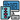# What is Asymptotic Notation?

If you are here for the first time and looking to start from scratch. Here's the order I have learned about Algorithms.

To properly learn something you have to start from the beginning. In this series of articles, We(yes me too) will learn about algorithms step by step. There are many books, tutorials, and blogs available on Algorithms, and it is often confusing to choose anyone from this large pool of resources. I was also confused but now I have decided that I will provide a summary of all the resources available and people don't have to look in different websites for every different topic.

We will be continuing our study on algorithms and discussing the analysis of algorithms.

This article, and the possible series I'll create from it, is meant for those who want to learn algorithms in an efficient way step by step and from a single resource or looking to revise concepts before a coding interview.

#### Goals

So, let's begin 🚀

#### Analysis of Algorithms

We discussed in last article that there can be many ways to solve a given problem but to decide which one is efficient, we use different types of analysis methods.

##### Types of Analysis

To analyze a given algorithm, we need to find how much time the algorithm takes for different inputs.

We represent the algorithm with multiple expressions:

• Case 1: Algorithm takes less time -> best-case
• Case 2: Algorithm takes more time -> worst-case

There are three types of analysis:

• Worst Case: Slowest time to complete for a given input -> Upper Bound
• Best Case: Fastest time to complete for a given input -> Lower Bound
• Average Case: Prediction about the running time of the algorithm by taking the average of total running time with the number of trials-> Average Time

Now, we can form a mathematical relation between these three types.

``Lower Bound <= Average Time <= Upper Bound``
##### Asymptotic Notation

After having relation between worst, average, best cases, we are ready to dive into different types of asymptotic notations, before that, we need to know about terms running time and rate of growth.

• Running Time

• The time an algorithm takes on a computer to run and that depends on the speed of the computer, the programming language, and the compiler that translates the program from the programming language into code that runs directly on the computer, among other factors.
• Rate of growth

• The rate at which running time increases as a function of input.

Some Commonly used growth rates in increasing order:

``````    - 1      Constant
- logn   Logarithmic
- n      Linear
- nlogn  Linear Logarithmic
- n^3    Cubic
- 2^n    Exponential``````

Let's understand by taking an algorithm, running on input size `n` with function `10n^2 + 100n + 100`. Here `10n^2` is larger than other terms, we can say that the running time of this algorithm grows as `n^2` and removing the `10` will also not make much difference.

Moving forward, we can imply that we need to simplify the function to keep the most important part and dropping less significant terms and the constant coefficients which we call asymptotic notation.

There are three types of asymptotic notation:

• Big-O Notation -> Upper Bounding Function
• Omega-Ω Notation -> Lower Bounding Function
• Theta-Θ Notation -> Order Function

We have reached at the end of this article, in the next article, we will be discussing Big-O notation.

Here are the next articles on Algorithms.

##### Did you find this post useful?

I would be really grateful if you let me know by sharing it on Twitter!

Follow me @ParthS0007 for more tech and blogging content :)